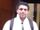## Solver in SAS - select rows that sum to a given value (with more than one constraint)

Small sample of data I have - for example:

Volume  Price   Tot. cost(vol*price)

100          5            500

200          6            1200

300          7             2100

400          8            3200

500          9            4500

1000        5             5000

I want to select the rows where: 1) Tot volume= 700 and 2) Tot. cost=5700.

In excel I use a binary variable that I multiply with Volume and Tot. cost and, after solving, it becomes 1 if the rows is selected and 0 otherwise.

I am not able to replicate this kind of solver in SAS. Thanks for your help!

1 ACCEPTED SOLUTION

Accepted Solutions

## Re: Solver in SAS - select rows that sum to a given value (with more than one constraint)

``````proc optmodel;
set OBS;
num volume {OBS};
num price {OBS};
num cost {i in OBS} = volume[i] * price[i];
read data have into OBS=[_N_] volume price;

var X {OBS} binary;
con TotalVolume:
sum {i in OBS} volume[i] * X[i] = 700;
con TotalCost:
sum {i in OBS} cost[i] * X[i] = 5700;

solve noobj;
create data want from [i]={i in OBS: X[i].sol > 0.5} volume price cost;
quit;``````
8 REPLIES 8

## Re: Solver in SAS - select rows that sum to a given value (with more than one constraint)

@sanjanko ,
You can try the following using the if-then-else construct. Similar to excel, here this will create a new data set which is an exact copy of what you have and add a new column with the logical condition. I am not sure if SAS would be ok with the period in your column name, If possible, I would rename that column in your source.

``````Data Want;
Set Have;
If Volume = 700 and Tot. cost = 5700 Then MyFlag = 1;
Else MyFlag = 0 ;
Run; ``````

## Re: Solver in SAS - select rows that sum to a given value (with more than one constraint)

I need to select the rows that sum up to Tot. Volume = 700. In this example, in order to obtain that Volume I need to select row 2 and row 5. All the others should not be selected. I am not able to do this selection of rows. In Solver Excel works, but I don't know how to reproduce it in SAS. Thanks!

## Re: Solver in SAS - select rows that sum to a given value (with more than one constraint)

@sanjanko ,
When you say select the rows, the equivalent in SAS that I am aware of is to create a subset of the original dataset which meets your conditions, you can do that using the if-then-else construct or where construct like below:

``````Data Want;
set have; *where have is the name of the dataset that you have;
Where Tot. Volume = 700;
Run;``````

## Re: Solver in SAS - select rows that sum to a given value (with more than one constraint)

I start from this data:

Volume Price Tot. cost(vol*price)

100 5 500

200 6 1200

300 7 2100

400 8 3200

500 9 4500

1000 5 5000

I need to obtain only that:

Volume Price Tot. cost(vol*price)

200 6 1200

500 9 4500

I only want to select these rows. The problem is that the sum of 200 and 500 gives me 700. It's not one unique row with 700,  but the sum. I need to tell SAS that it has to select rows that sum up to a certain value. This should be done with: PROC OPTMODEL; just my code does't work. Thanks.

## Re: Solver in SAS - select rows that sum to a given value (with more than one constraint)

What code? You haven't shown any code?
You're expecting exactly the values 700 and 5700?novinosrin
Tourmaline | Level 20

## Re: Solver in SAS - select rows that sum to a given value (with more than one constraint)

@sanjanko   See if this works

``````data have;
input Volume  Price   Tot  ;
cards;
100          5            500
200          6            1200
300          7             2100
400          8            3200
500          9            4500
1000        5             5000
;

proc sql;
create table want(drop=s v) as
select a.* ,b.volume as v,sum(a.volume,b.volume)=700 as s
from have a, have(keep=volume) b
group by s
having (min(a.volume)=a.volume and s) or (min(b.volume)=b.volume and s );
quit;
``````

## Re: Solver in SAS - select rows that sum to a given value (with more than one constraint)

``````proc optmodel;
set OBS;
num volume {OBS};
num price {OBS};
num cost {i in OBS} = volume[i] * price[i];
read data have into OBS=[_N_] volume price;

var X {OBS} binary;
con TotalVolume:
sum {i in OBS} volume[i] * X[i] = 700;
con TotalCost:
sum {i in OBS} cost[i] * X[i] = 5700;

solve noobj;
create data want from [i]={i in OBS: X[i].sol > 0.5} volume price cost;
quit;``````

## Re: Solver in SAS - select rows that sum to a given value (with more than one constraint)

Thanks a lot! That's exactly what I need!

Discussion stats
• 8 replies
• 987 views
• 0 likes
• 5 in conversation